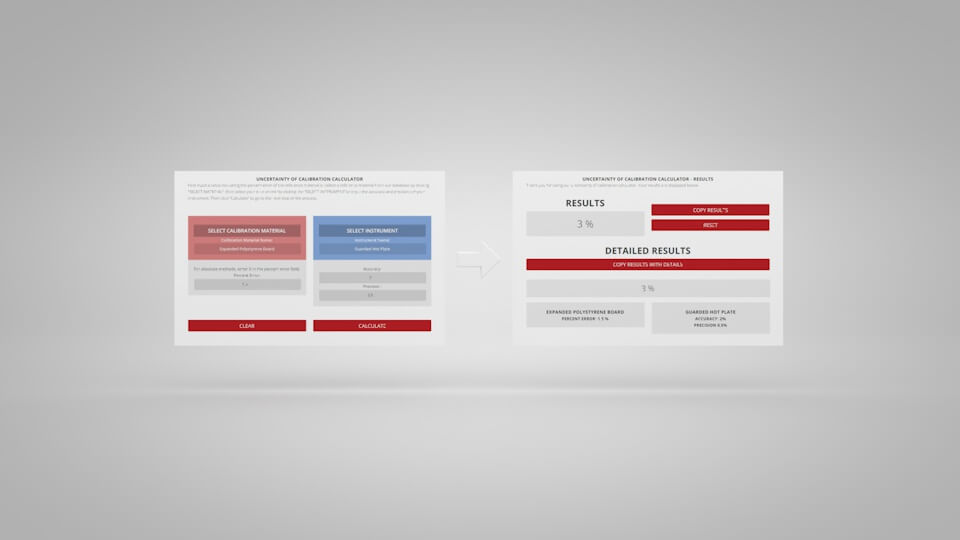# Uncertainty Calculator for Thermal Conductivity Instruments

Thermal Conductivity Instruments are classified into two main categories; absolute and comparative. For calculation of uncertainty (%) for absolute methods, a simple composite error calculation from accuracy and precision is possible. For calculation of uncertainty (%) for comparative methods, addition of percent error of the reference material must be considered. Comparative can be further broken down into two categories, single-off-set and secondary calibration.

This uncertainty calculator tool for comparative methods is only valid for a single-off-set calibration, comparative methods requiring a secondary calibration, which involve the use of multiple reference materials have a much higher composite uncertainty.

The uncertainty of an arbitrary function $$R$$ with any number of variables $$(X,Y\ldots)$$ can be expressed by taking the root of the
sum of the squares of the partial derivatives of $$R$$ with respect to each variable multiplied by the uncertainty in that variable.

$R=R(X,Y,\ldots)$

$\delta{R}=\sqrt{\Big ( \frac{\partial R}{\partial X}\cdot \delta X \Big) ^{2} + \Big ( \frac{\partial R}{\partial Y}\cdot \delta Y \Big) ^{2} + \ldots}$

### For uncertainty calculation, there are a few variables to consider:

• All methods: The measure of accuracy (%) and measure of precision (%).
• Comparative Methods: Percent error (%) of reference material.
• Absolute Methods: For absolute methods, enter 0 in the percent error field.## Uncertainty of Calibration Calculator Blog Post

Want to learn more about the uncertainty of calibration calculator? Check out the UNCERTAINTY OF CALIBRATION CALCULATOR blog post to learn how it works.

## UNCERTAINTY OF CALIBRATION CALCULATOR

First input a value indicating the percent error of the reference material or select a reference material from our database by clicking “SELECT MATERIAL”, then select your instrument by clicking the “SELECT INSTRUMENT”or input the accuracy and precision of your instrument. Then click “Calculate” to go to the next step of the process.

Instrument Name:
Accuracy: Precision:
Calibration Material Name:
For absolute methods, enter 0 in the percent error field. Percent Error:

Reference:

1. "Reference materials for thermal transport properties measurements" - Springer.com
2. Taylor, J. An Introduction to Error Analysis: The Study of Uncertainties in Physical Measurements. University Science Books; 2nd edition (August 1, 1996). Available at amazon.com